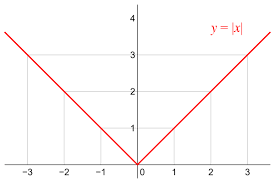# Java Program To Calculate Modulus | Mod Java

Java Program To Calculate Modulus – In this article, we will explain the multitude of methods to calculate the modulus of a number in Java Programming. Suitable examples and sample programs have been included in order to make you understand simply. The compiler has also been added so that you can execute the programs yourself.

The methods used in this article are as follows to calculate Java Modulus

• Using Standard Method
• Using Scanner Class
• Using Command Line Arguments
• Using Static Method
• Using Separate Class

The mod of a number can be regarded as the absolute value of any given number. It plays an important role in pretty much all sectors of mathematics.For example,

The modulus of -22 will be represented as

| -22 | = 22.

As you can see in the image above, it is the graphical representation of the mod function. The absolute value is always positive no matter what.

Thus, the methods used to calculate the mod of a number in Java Programming are as follows:

## Java Mod Code

In here, the entire program is written within the main method itself.

Our input is a predetermined integer number and our output is an integer as well which is the mod of the input number.

There are two ways this can be achieved in Java. They are:-

1. We can directly make use of the absolute method or the abs method from the Math package which consists of a predefined function to do this.
2. All one has to do is, use Math.abs(int number) and you get the output as the mod of the integer given as argument.
3. Another way is to first check whether the number is positive or negative.
4. If the number is negative i.e., if(number<0) then we  multiply number with “-1”.
5. This is because, a negative number multiplied with -1 gives a positive number of the same digits which is nothing but the mod. Else the number remains the same because, the mod of positive number is the number itself.

Output:

### Java Modulus/Mod using Scanner class

In the above method, we have seen that the integer to find mod is predetermined and given at compile time in the code itself and if we need the mod of another number then the change must be made in the code. This kind of method is not efficient at all.

Hence we can make use of the scanner class in java.

The scanner class of Java accepts inputs of any primitive data type at run time itself. This makes things a lot more convenient. So, after taking the input using scanner class the same logic as above can be applied here.

Output1:

#### Using Command Line Arguments

Command line arguments in Java are the arguments that are passed while running the run command just after the name of the Java program separated by space between them.

Similarly here, while running Java code a single argument whose mod is to be found can be given with the run command separated with the space. This argument (arg) is converted into integer using parseInt() as follows:

After this, the same logic can be made use of to find out the mod of this integer.

Output:

Using Static Method

Static method is a method that makes use of static variables and performs the actions as written in it. We can make use of static method just to differentiate the inputs and logic from each other for better understanding.

Therefore, in the main method, inputs can be taken in with the use of Scanner Class.

These inputs can then be sent as parameters of the static method.

A separate static method (modCal) can be written which consists of any of the two logic written above and return the mod of the integer which can then be displayed in the console screen.

x

## Plus Star Pattern Java Program | Patterns

Java program to print plus star pattern program – We have written the below print/draw ...